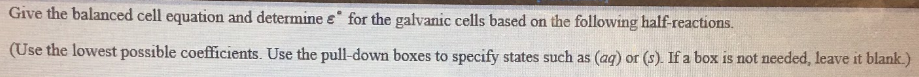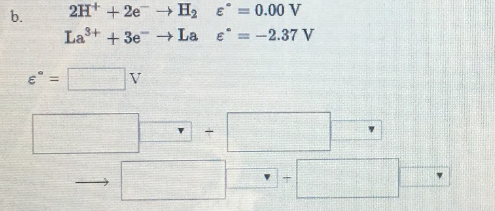# Give the balanced cell equation and determine E° for the galvanic cells based on the following half-reactions. (Use the lowest possible coefficients. Use the pull-down boxes to specify states such as (aq) or (s). If a box is not needed, leave it blank.) b. 2H^+ + 2e^- → H2 E° = 0.00 V La^3+ + 3e^- → La E° = -2.37 V